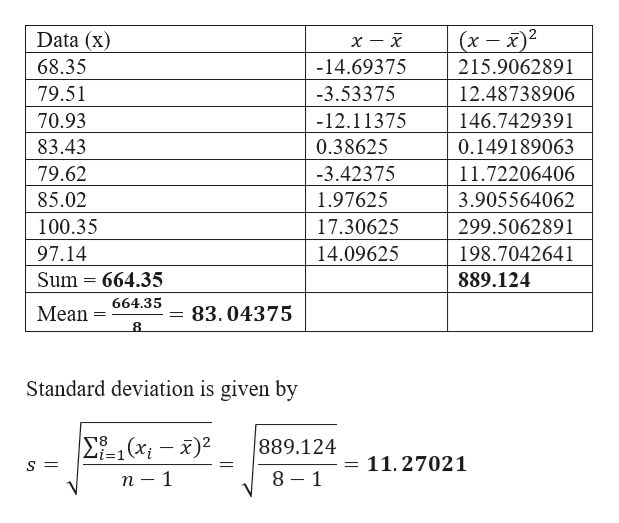Question
401 views
check_circle

Step 1

Given datahelp_outlineImage Transcriptionclose(x - 2 Data (x) 68.35 -14.69375 215.9062891 79.51 -3.53375 12.48738906 70.93 -12.11375 146.7429391 83.43 0.38625 0.149189063 79.62 -3.42375 11.72206406 85.02 1.97625 3.905564062 100.35 17.30625 299.5062891 97.14 14.09625 198.7042641 Sum 664.35 889.124 664.35 Mean = 83.04375 Standard deviation is given by Σ-1 (x - )2 889.124 11.27021 8 1 п — 1 fullscreen
Step 2

b)

95% confidence interval is given by

The 100 (1 – α) % confidence interval for the population mean, μ, for given sample standard deviation, s is: ( – (/2; n – 1­) (s/√n),  + (/2; n – 1) (s/√n)).

Here, n is the sample size,  is the sample mean, and /2; n – 1 is the critical value of the t-distribution with (n – 1) degrees of freedom, above which, 100 (α/2) % or α/2 proportion of the observations lie.

The t-distribution is used, because the population standard deviation is unknown and the sample standard deviation is being used as a substitute.

Calculation:

Here, n = 8;  = 83.04375; s = 11.27021.

Therefore, degrees of freedom = 8 – 1 = 7.

Again, 100 (1 – α) % = 95% = 0.95.

Thus, α = 0.05.

From the Excel formula: =T.I...

### Want to see the full answer?

See Solution

#### Want to see this answer and more?

Solutions are written by subject experts who are available 24/7. Questions are typically answered within 1 hour.*

See Solution
*Response times may vary by subject and question.
Tagged in

### Other